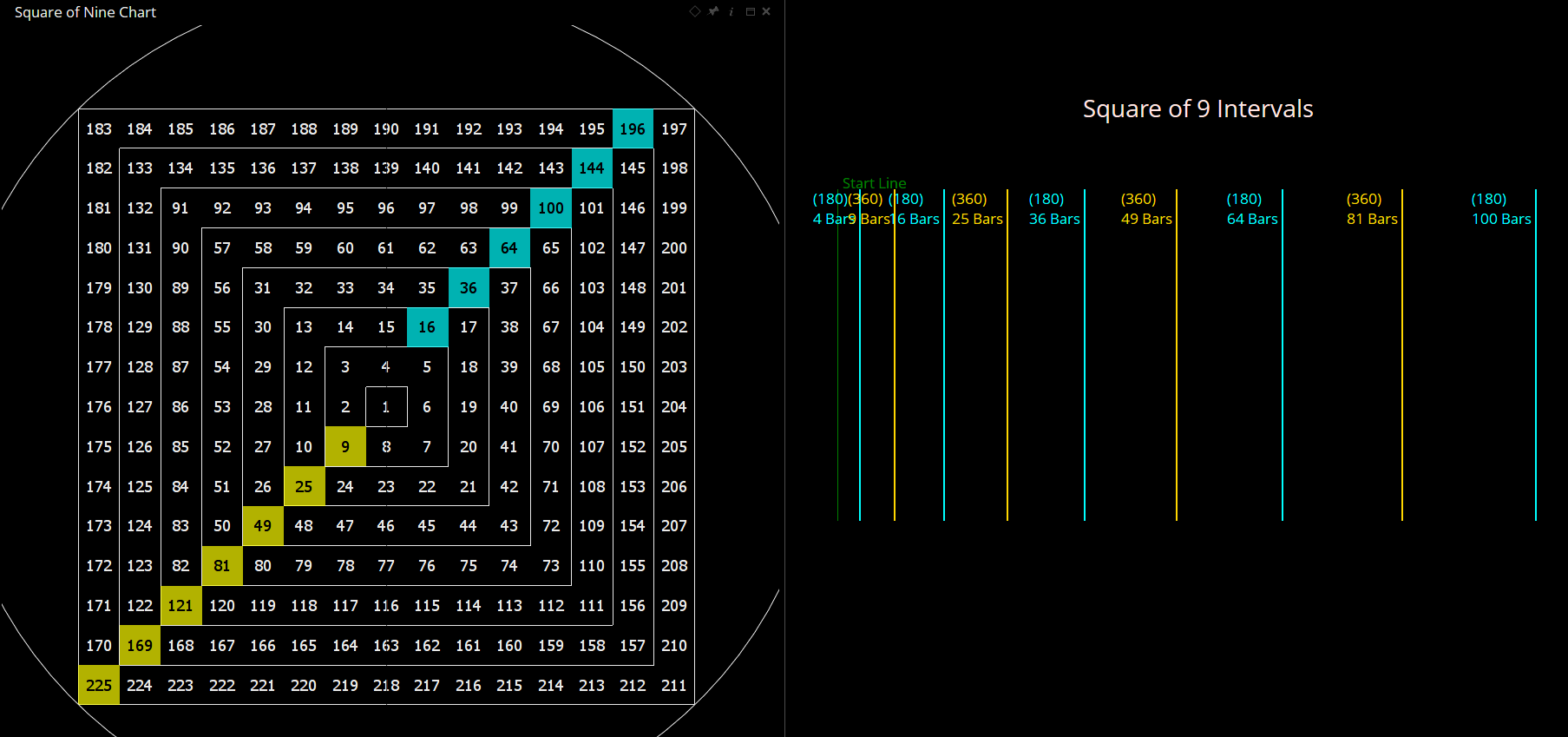Select Page

# Reply To: Square of Nine

Optuma Forums Optuma General Discussion Square of Nine Reply To: Square of Nine

#63251

Hi Thomas,

It’s because of the way the angles are calculated from a starting point of 1.

360° around the wheel is calculated as (√(n)+2)²

so where n=1:

(1+2)² = 9

180° around the wheel is calculated as (√(n)+1

Therefore, if 9 is the 1st 360° level, the tool calculates 180° from there as follows:

(√(9)+1)² = 16

The next 360° value around the square from 9 is calculated as (3+2)² = 25.

Keep repeating the calculations and you get the squares of the odd numbers (9, 25, 49, 81, etc) expanding to the bottom left, with the even numbers (16, 36, 64, 100, etc) appearing opposite ie 180° heading top-right.

I hope that makes sense.###### 1 user thanked author for this post.Thomas